Courses

# Test: Power Systems- 2

## 10 Questions MCQ Test RRB JE for Electrical Engineering | Test: Power Systems- 2

Description
This mock test of Test: Power Systems- 2 for Electrical Engineering (EE) helps you for every Electrical Engineering (EE) entrance exam. This contains 10 Multiple Choice Questions for Electrical Engineering (EE) Test: Power Systems- 2 (mcq) to study with solutions a complete question bank. The solved questions answers in this Test: Power Systems- 2 quiz give you a good mix of easy questions and tough questions. Electrical Engineering (EE) students definitely take this Test: Power Systems- 2 exercise for a better result in the exam. You can find other Test: Power Systems- 2 extra questions, long questions & short questions for Electrical Engineering (EE) on EduRev as well by searching above.
QUESTION: 1

### When series reactor is used in generating stations, the fault current magnitude is

Solution:

Fault current is inversely proportional to reactance of the network. Therefore with series reactor, the fault current magnitude is reduced. It is preferred in the generating stations in some special applications for limiting the current flowing through the low MVA rating circuit breakers.

*Answer can only contain numeric values
QUESTION: 2

### A surge of 200 kV travels along an overhead line towards its junction with a cable. The surge impedance for the overhead line and cable are 450 Ω and 60 Ω respectively. The magnitude of the surge transmitted through cable is – (in kV)

Solution: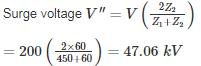QUESTION: 3

### Ds is the GMR of each sub-conductor of a four sub-conductor bundle conductor and dd is the bundle spacing. What is the GMR of the equivalent single conductor?

Solution: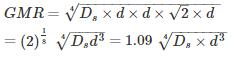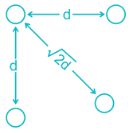*Answer can only contain numeric values
QUESTION: 4

A transmission line having open circuit impedance and short circuit impedance parameters are respectively  Zoc = 900∠ − 30, ZSC = 400∠30∘ , Find the characteristic impedance of the line – (in Ω)

Solution: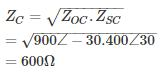QUESTION: 5

A three-phase, 50 Hz transmission line of length 120 km has a capacitance of 0.05/π μF/km It is represented as a π – model. The shunt admittance at each end of the transmission line will be

Solution:

In π model, the shunt admittance at each end of the line is Y/2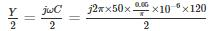= j300 × 10−6

= 300 × 10−6 ∠90mho

*Answer can only contain numeric values
QUESTION: 6

A three-phase, 22 kV line feeds a per – phase load of 15 MW. If the impedance of the Line is Z = j 10Ω, then the value of load angle to maintain a line voltage of 22 kV at the load is_____ (in degrees)

Solution:

Per phase sending and receiving end voltage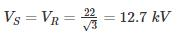We know that for a loss – less line (R = 0) real power is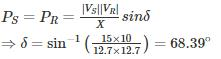*Answer can only contain numeric values
QUESTION: 7

What is the maximum length (in km) for a single phase transmission line having a copper conductor of 0.8 cm2 cross-section over which 250 kW at unity power factor and at 3 kV are to be delivered?

The efficiency of transmission is 90%. The specific resistance as 2 μΩ-cm.

Solution:

Receiving end power = 250 kW

Efficiency of transmission = 90%
Sending end power =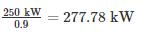Line losses = 277.78− 250 = 27.78kW
Line current, I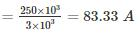Let R be the resistance of one conductor.

Line losses = 2I2R
⇒27.78 × 10= 2(83.33)2R
⇒ R = 2 Ω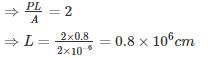= 8 km

QUESTION: 8

Two overhead Lines ‘P’ and ‘Q’ are connected in parallel to supply a load of 10 MW at 0.8 pf lagging. The resistance and reactance of line ‘P’ are 3 Ω and 4 Ω, respectively and of the Line, ‘Q’ is 4 Ω and 3 Ω respectively. The power supplied by the ‘P’ is

Solution:

Impedance of Line P, ZP = 3 + j4 = 5∠53.13° Ω

Impedance of Line Q, ZQ = 4 + j3 = 5∠36.86° Ω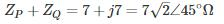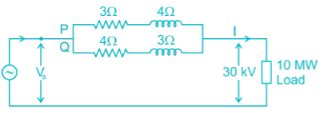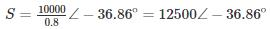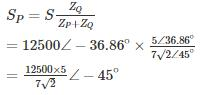= 6313.45 kVA at pf 0.708

Power supplied by Line P,

P = 6313.45 × 0.707 = 4.46 MW

*Answer can only contain numeric values
QUESTION: 9

The load on an installation is 600 kW, 0.8 lagging p.f. which works for 3000 hours per annum. The tariff is Rs. 100 per KVA plus 20 Paise per kWh. If the power factor is improved to 0.9 lagging by means of loss of free capacitors costing Rs. 60 per kVAR, calculate the annual cost saving (in Rupees). Allow 10% per annum for interest and depreciation on capacitors.

Solution:

D = 600 kW

Cosϕ1 = 0.8

Cosϕ2 = 0.9

= P (tanϕ1 – tanϕ2)

= 600 (tan (cos-1(0.8)) – tan (cos-1 (0.9)))

= 159.41 KVAR

Annual cost before p.f. correction

Maximum KVA demand = 600/0.8=750

KVA demand charges = 750 × 100 = 75,000

Units consumed per annum = 600 × 3000 = 1800000 kWh

Energy charges per year = 0.2 × 1800000 = 360000

Total annual cost = 75000 + 360000 = 435000

Annual cost after p.f. correction

Maximum KVA demand =600/0.9 = 666.67

KVA demand charges = 666.67 × 100 = 66, 667

Energy charges = 360000

Capital cost of capacitors = 60 × 159.41 = 9564.6

Annual interest and depreciation = 0.1 × 9564.6 = 956.46

Total annual cost = 66667 + 360000 + 956.46 = 427623. 46

Annual saving = 435000 – 427623.46 = 7376.54

QUESTION: 10

Two generators rated 250 MW and 400 MW are operating in parallel. The droop characteristics of the governors are 4% and 6% respectively.if a load of 450 MW is share between them. What will be the system frequency? Assume nominal system frequency is 60 Hz and no governing action.

Solution:

Let, Load on generator 1 = x MW

Load on generator 2 = (450 – x) MW

Reduction in frequency = Δf

Now,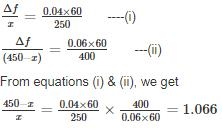∴ x = 217.81 MW (load on generator 1)

450 – x = 232.18 MW (load on generator 2)

and Δf = 2.09Hz

∴ System frequency = (60 – 2.09) = 57.909 Hz.